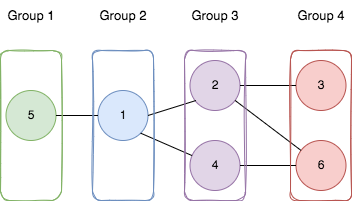2493. Divide Nodes Into the Maximum Number of Groups
Hard
312
16

You are given a positive integer `n` representing the number of nodes in an undirected graph. The nodes are labeled from `1` to `n`.

You are also given a 2D integer array `edges`, where `edges[i] = [ai, bi]` indicates that there is a bidirectional edge between nodes `ai` and `bi`. Notice that the given graph may be disconnected.

Divide the nodes of the graph into `m` groups (1-indexed) such that:

• Each node in the graph belongs to exactly one group.
• For every pair of nodes in the graph that are connected by an edge `[ai, bi]`, if `ai` belongs to the group with index `x`, and `bi` belongs to the group with index `y`, then `|y - x| = 1`.

Return the maximum number of groups (i.e., maximum `m`) into which you can divide the nodes. Return `-1` if it is impossible to group the nodes with the given conditions.

Example 1:```Input: n = 6, edges = [[1,2],[1,4],[1,5],[2,6],[2,3],[4,6]]
Output: 4
Explanation: As shown in the image we:
- Add node 5 to the first group.
- Add node 1 to the second group.
- Add nodes 2 and 4 to the third group.
- Add nodes 3 and 6 to the fourth group.
We can see that every edge is satisfied.
It can be shown that that if we create a fifth group and move any node from the third or fourth group to it, at least on of the edges will not be satisfied.
```

Example 2:

```Input: n = 3, edges = [[1,2],[2,3],[3,1]]
Output: -1
Explanation: If we add node 1 to the first group, node 2 to the second group, and node 3 to the third group to satisfy the first two edges, we can see that the third edge will not be satisfied.
It can be shown that no grouping is possible.
```

Constraints:

• `1 <= n <= 500`
• `1 <= edges.length <= 104`
• `edges[i].length == 2`
• `1 <= ai, bi <= n`
• `ai != bi`
• There is at most one edge between any pair of vertices.
Accepted
5.3K
Submissions
14.4K
Acceptance Rate
37.0%

Seen this question in a real interview before?
1/4
Yes
No

Discussion (0)

Related Topics